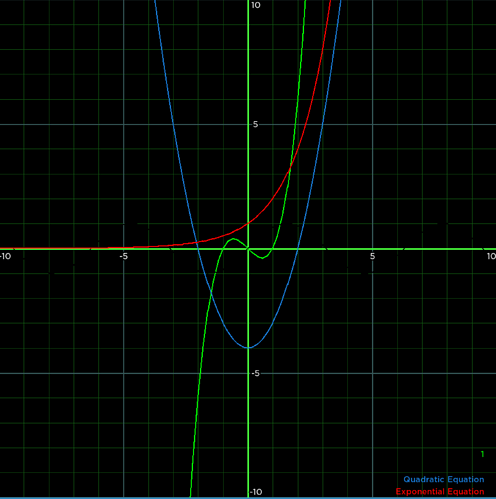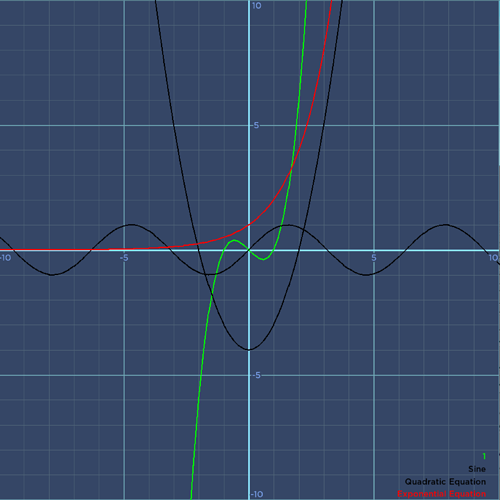# Graphit || Display and compare your functions• Graphit is a module that allows you to easily display functions as a 2d element

Usage

• This module currently only has one function, used for graphing;
This function has 4 parameters for customization
``````module.Graph(Frame, Size, Functions, Theme)
``````
• Frame

The frame parameter is used to get the frame the function will be in. I recommend this frame to contain a `UIAspectRatioConstraint` object, other wise the graphs will be squished or stretched depending on the length and width of the frame.

• Size

This parameter alters the zoom of a the graph, if the size is set to 10, the graph will range from -10 to 10 on both axis

• Functions

The functions parameter is a table, containing as many tables, of tables.

Here is an example of this parameter

``````local functionsTable = {
['Quadratic Equation'] = {function(x) return x^2 - 4 end}, -- will assign a random color to this quadratic
['Exponential Equation'] = {function(x) return 2^x end,Color3.fromRGB(255,0,0)}, -- will graph a red, exponential function
['Sine'] = {function(x) return math.sin(x) end,Color3.fromRGB(0,0,0)}, -- will graph a black, sine wave
{function(x) return x^3 - x end,Color3.fromRGB(0,255,0)}, -- will graph a green cubic function under the name of a number
}
``````
• Theme

The theme parameter is an optional parameter. This parameter is a table in the form of this

``````--Default theme
local theme = {
['Background'] = Color3.fromRGB(255, 255, 255),
['GridLines'] = {
['Axis'] = Color3.fromRGB(0, 0, 0),
['Large'] = Color3.fromRGB(173, 173, 173),
['Small'] = Color3.fromRGB(222,222,222)
}
}
``````

After all these settings are applied, the final graph looks like this

Sample

``````local graph = require(game.ReplicatedStorage.Graph).Graph
local frame = script.Parent.Frame
local size = 10
local functionsTable = {
['Quadratic Equation'] = {function(x) return x^2 - 4 end}, -- will assign a random color to this quadratic
['Exponential Equation'] = {function(x) return 2^x end,Color3.fromRGB(255,0,0)}, -- will graph a red, exponential function
['Sine'] = {function(x) return math.sin(x) end,Color3.fromRGB(0,0,0)}, -- will graph a black, sine wave
{function(x) return x^3 - x end,Color3.fromRGB(0,255,0)}, -- will graph a green cubic function under the name of a number
}

local theme = {
['Background'] = Color3.fromRGB(255, 255, 255),
['GridLines'] = {
['Axis'] = Color3.fromRGB(0, 0, 0),
['Large'] = Color3.fromRGB(173, 173, 173),
['Small'] = Color3.fromRGB(222,222,222)
}
}
graph(frame, size, functionsTable, theme)
``````

• The ability to make functions visible and invisible when you interact with the names

• The capability to change the theme;
Note: If no theme parameter is specified, the module will create its own theme based on the color of the frame. In most cases this theme isnt favorable. Unless your frame is white, resort to making your own theme.
Images

## Hacker

``````local theme = {
['Background'] = Color3.fromRGB(0, 0, 0),
['GridLines'] = {
['Axis'] = Color3.fromRGB(73, 255, 60),
['Large'] = Color3.fromRGB(54, 89, 89),
['Small'] = Color3.fromRGB(17, 83, 17)
}
}
``````

##image1805×1816 34.5 KB Bluish

``````local theme = {
['Background'] = Color3.fromRGB(53, 70, 102),
['GridLines'] = {
['Axis'] = Color3.fromRGB(140, 230, 255),
['Large'] = Color3.fromRGB(108, 162, 177),
['Small'] = Color3.fromRGB(80, 101, 120)
}
}
``````

##image1813×1813 47.5 KB Dark

``````local theme = {
['Background'] = Color3.fromRGB(40, 40, 40),
['GridLines'] = {
['Axis'] = Color3.fromRGB(255, 255, 255),
['Large'] = Color3.fromRGB(100,100,100),
['Small'] = Color3.fromRGB(70, 70, 70)
}
}
``````

Message from Developer

I am aware that there’s a better, (probably more efficient) graph module by boatbomber. The module does have a lot more ways of usage in games as appose to the one I’ve created. However i created this mainly for myself and anyone crazy about math who wants to visualize their functions.

### If you find any bugs or have any suggestions (which I’m sure you will) please report them to me in the replies

Credits

Default UI inspired by Desmos
@XxSalmonloaferxX2 for helping me get an incrementation system for the graph, again similar to the one desmos has
@Artoshia for the segment between two points function

11 Likes

holy ####, this is amazing! thank you for your creation!

1 Like

Very useful, thanks alot for this! (:

1 Like